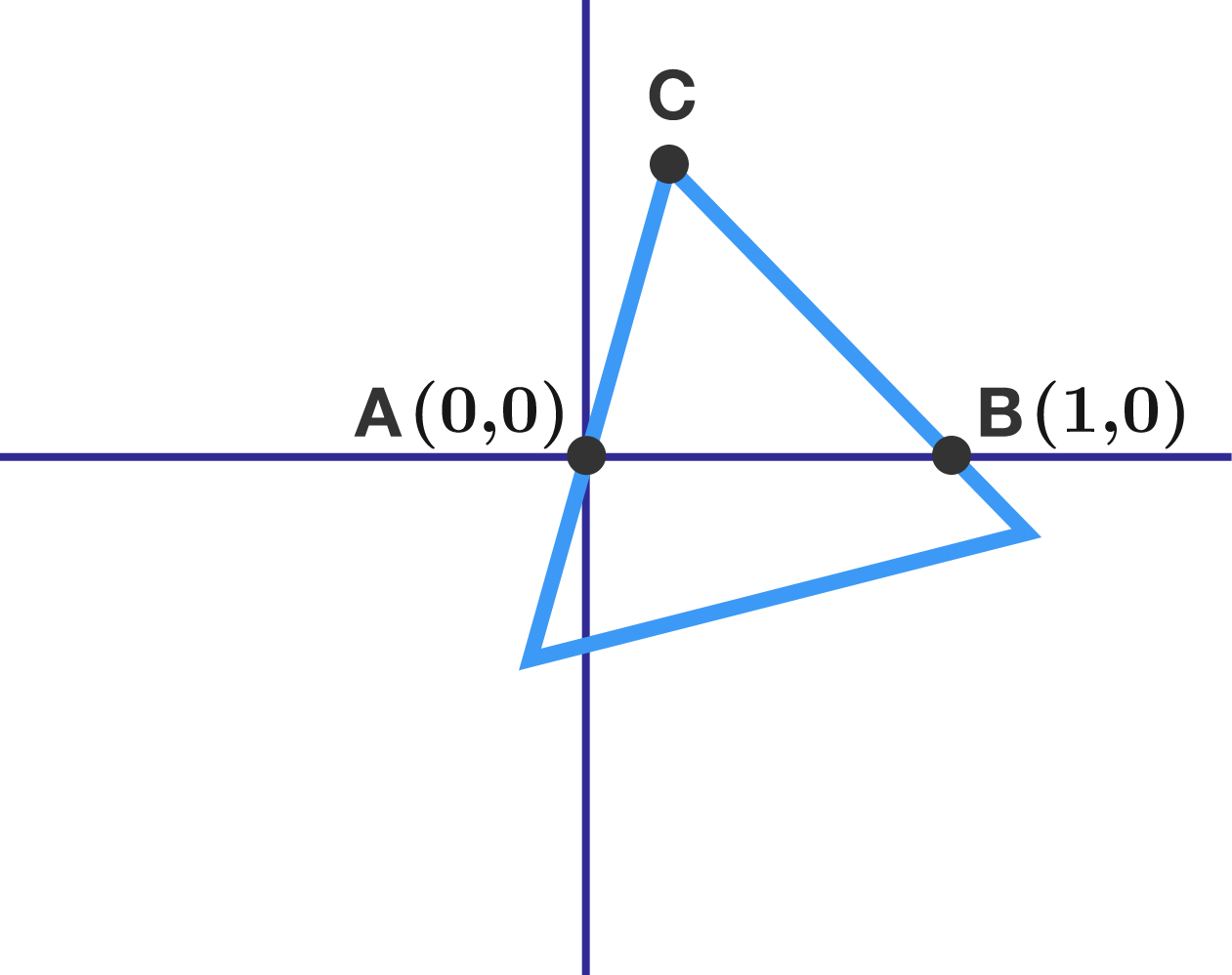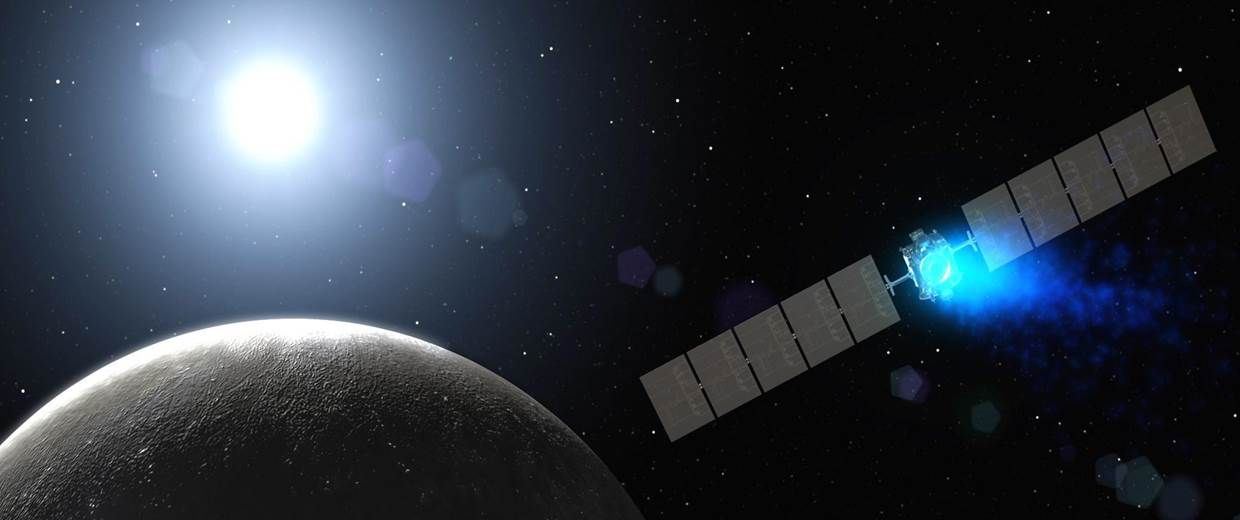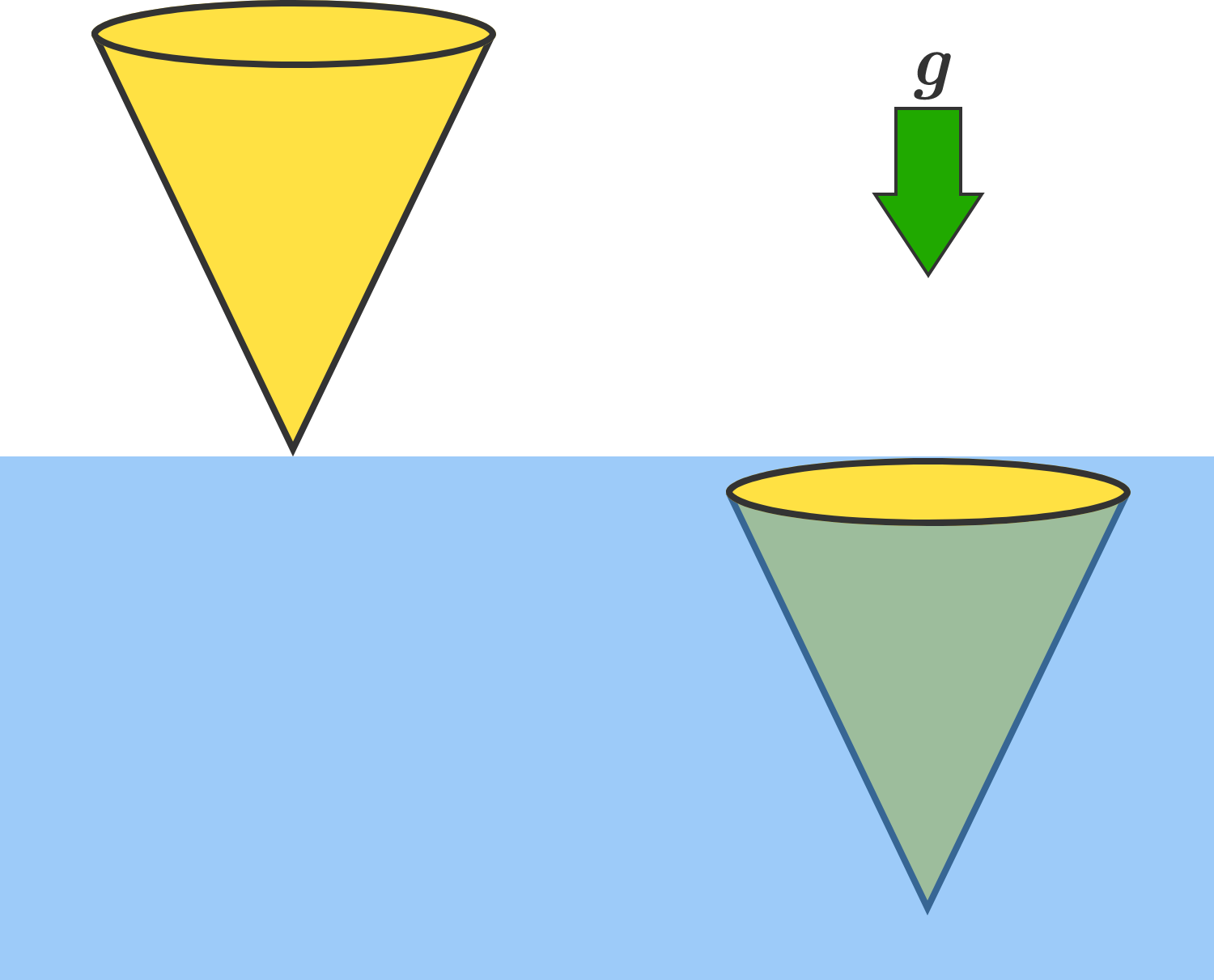# Problems of the Week

Contribute a problem

$(x+y)(x+z)(y+z) = 2017^n$

What is the minimum value of $n$ such that there exist positive integers $x,y,$ and $z$ satisfying the equation above?An equilateral triangle

• has two of its vertices below the $x$-axis,
• has the third vertex $C$ above the $x$-axis, and
• contains $A=(0,0)$ and $B=(1,0)$ on its sides.

How long is the path traced out by all possible points $C,$ to two decimal places?

If you think the path is infinitely long, provide 99999 as your answer. Or, if you think all the possible locations of $C$ represent an area rather than a path, submit 99998 as your answer.

An interplanetary probe speeding in deep space suddenly needs to make a left turn.It is presently traveling at $v= \SI[per-mode=symbol]{20000}{\meter\per\second}$, and needs to make a quarter circle turn, continuing at the same tangential speed. It is equipped with an ion thruster rocket capable of ejecting ions at $V= \SI[per-mode=symbol]{50000}{\meter\per\second}$. Moreover, it is a very advanced model that gets its ions from outer space so that the weight of the probe remains unchanged during its turn.

It takes a certain amount of total energy $E$ to propel the probe around this quarter circle turn. If the kinetic energy of the probe is $T=\frac{1}{2}m{v}^{2}$ (which remains unchanged throughout), what is the ratio $\dfrac{E}{T}?$ Submit your answer to 3 decimal places.


Details and Assumptions:

• Assume that no energy is gained or lost in the probe's acquisition of interplanetary ions before they are ejected by the ion thruster engine.
• Assume that the probe undergoes rotation during the turn so that the ion thrust always points outward from the center of the turn, again with no energy expended for this rotation.
• Assume that there are no gravitational masses nearby.A solid right circular cone of uniform mass density is initially at rest above a body of water, so that its vertex is just touching the water's surface with its axis of symmetry along the vertical.

Now, the cone falls into the water, and has zero speed at the instant it becomes fully submerged. What is the ratio of the density of the cone to the density of the water? Submit your answer to 2 decimal places.


Details and Assumptions:

• There is an ambient downward gravitational field.
• Assume that the buoyant force is the only force exerted by the water on the cone.

$a^3+b^3+3\big(a^2+b^2\big)=700(a+b)^2$

Let $a$ and $b$ be rational numbers such that $a \ge b,$ their sum is an integer, and the equation above is fulfilled. Find all solutions $(a, b)$ and enter your answer as $\sum (a+b)$.

×